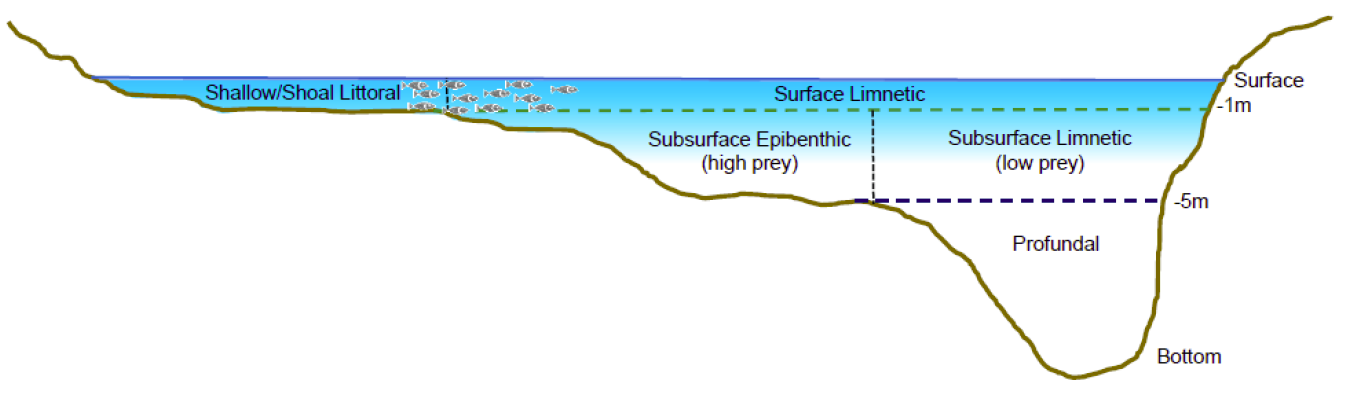The quality of physical habitat for juvenile salmonids is defined according to specifications outlined by the Water Quality Parameter and Habitat Productivity Technical Group. Habitat quality is assessed in terms of temperature, salinity and dissolved oxygen thresholds, with four categories defined for each variable.

#### Temperature:

optimal growth positive growth no/negative growth unsuitable
$$14-18^\circ\text{C}$$ $$< 14^\circ\text{C}$$ or $$18-21^\circ\text{C}$$ $$21-25^\circ\text{C}$$ $$> 25^\circ\text{C}$$

#### Dissolved Oxygen:

minimal impairment some impairment severe impairment unsuitable
$$> 6 \text{ mg/L}$$ $$4-6 \text{ mg/L}$$ $$3-4 \text{ mg/L}$$ $$< 3 \text{ mg/L}$$

#### Salinity:

freshwater isotonic brackish marine
$$< 10 \text{ ppt}$$ $$10-15 \text{ ppt}$$ $$15-28 \text{ ppt}$$ $$> 28 \text{ ppt}$$
Low energy demand negligible energy demand high energy demand (freshwater-acclimated) unsuitable (freshwater acclimated)
low energy demand (saltwater-aclimated) moderate energy demand (saltwater-aclimated)

### Overall Habitat

To assess the overall habitat quality of a region, the individual ratings are into the 9 categories defined below.

category Description
Optimal optimal or positive growth (T); minimal impairment (DO); Low or negligible energy demand (S)
Growth limited no/negative growth (T); minimal impairment (DO); Low or negligible energy demand (S)
Impaired optimal or positive growth (T); some or severe impairment (DO); Low or negligible energy demand (S)
Energy demanding optimal or positive growth (T); minimal impairment (DO); moderate or high energy demand (S)
Growth limited, impaired no/negative growth (T); some or severe impairment (DO); Low or negligible energy demand (S)
Growth limited, energy demanding no/negative growth (T); minimal impairment (DO); moderate or high energy demand (S)
impaired, energy demanding optimal or positive growth (T); some or severe impairment (DO); moderate or high energy demand (S)
Growth limited, impaired, energy demanding no/negative growth (T); some or severe impairment (DO); moderate or high energy demand (S)
Unsuitable any one of: unsuitable (T); unsuitable (S); unsuitable (DO)

### Depth Zone Definitions

Habitat quality can also be assessed based on water depth, which has important implications for foraging, shelter and predation risk. The Water Quality Parameter and Habitat Productivity Technical Group has defined a separate set of habitat categories that assess habitat in terms of water depth.Depth categories

Category Description Criteria
Littoral (Shallow/shoal) High prey availability, high avian predation risk, low aquatic predation risk $$depth < 1\text{m}$$ and $$\max{(depth)} < 1\text{m}$$
Surface Limnetic Low prey availability, high avian predation risk, high aquatic predation risk $$depth < 1\text{m}$$ and $$\max{(depth)} \geq 1\text{m}$$
Epibenthic High prey availability, low avian predation risk, moderate aquatic predation risk $$1\text{m} \leq depth \leq 5\text{m}$$ and $$\max{(depth)} \leq 5$$
Subsurface Limnetic Low prey availability, low avian predation risk, moderate aquatic predation risk $$1\text{m} \leq depth \leq 5\text{m}$$ and $$\max{(depth)} > 5$$
Profundal Low prey availability, no predation risk $$depth > 5\text{m}$$